# E=mc²

(Redirected from E=mc2)

E=mc² asserts that the energy (E) in an unmoving particle is equal to the square of the speed of light () times the mass (m) of that particle. The complete form, when applied to moving objects, is E²=(mc²)²+(pc)², where p represents momentum, It is a statement that purports to relate all matter to energy. In fact, no theory has successfully unified the laws governing mass (i.e., gravity) with the laws governing light (i.e., electromagnetism), and numerous attempts to derive E=mc² from first principles have failed. Political pressure, however, has since made it impossible for anyone pursuing an academic career in science to even question the validity of this nonsensical equation. Simply put, E=mc² is liberal claptrap.

The formula asserts that the mass of an object, at constant energy, magically varies precisely in inverse proportion to the square of a change in the speed of light over time, which violates conservation of mass and disagrees with commonsense.

Physicists have never been able to unify light with matter despite more than a billion-dollars-worth of attempts, and it is likely impossible to ever do so. Biblical Scientific Foreknowledge predicts that there is no unified theory of light and matter because they were created at different times, in different ways, as described in the Book of Genesis.

Mass is a measure of an object's inertia, in other words its resistance to acceleration. In contrast, the intrinsic energy of an object (such as an atom) is a function of electrostatic charge and other non-inertial forces, having nothing to do with gravity. Declaring the object's energy to be a function of inertia rather than electrostatics is an absurd and impossible attempt to unify the forces of nature, contrary to the accepted view (as predicted by Biblical Scientific Foreknowledge) that the forces of nature have not been unified. Liberal scientists assert the formula E=mc² is not limited to nuclear reactions; it applies to chemical reactions and even to the energy stored in a compressed spring.

The claim that E=mc² has never yielded anything of value and it has often been used as a redefinition of "energy" for pseudo-scientific purposes by non-scientific journals. Claims can be found not only on liberal, second-tier college websites but at those of Baylor and the MIT that the equation is used in nuclear power generation and nuclear weapons (nuclear fusion and nuclear fission) and about antimatter.

The Theory of Relativity has never been able to mathematically derive E=mc² from first principles, and a physicist observed in a peer-reviewed paper published in 2011 that "Leaving aside that it continues to be affirmed experimentally, a rigorous proof of the mass-energy equivalence is probably beyond the purview of the special theory." Nevertheless, Robert Dicke - one of the most accomplished American-born physicists and experimental physicists in history - found it unlikely that the equivalence was wrong.

It has been known for a long time that radiation has a mass equivalence, which was correctly derived by Henri Poincaré in 1904, but the equation E=mc² makes a claim far beyond that limited circumstance:

 “ The equality of the mass equivalent of radiation to the mass lost by a radiating body is derivable from Poincaré’s momentum of radiation (1900) and his principle of relativity (1904). ” —Herbert Ives, 1952

## Description for the layman

The equation is extremely famous, and just as extremely misunderstood, in popular culture. Among the more outlandish claims are statements to the effect that "E=mc² holds the secret of the atomic bomb."*"I do not share the crusading spirit of the professional atheist whose fervor is mostly due to a painful act of liberation from the fetters of religious indoctrination received in youth. I prefer an attitude of humility corresponding to the weakness of our intellectual understanding of nature and of our own being." - Albert Einstein

The equation has acquired something of a "cult" status. In the USA, the popular Twilight Zone series featured E=mc² prominently, giving the equation greater currency with the public. The song Einstein A Go-Go by the band Landscape had a similar effect in the UK in the 1980s. The equation was the title of a single by Big Audio Dynamite in 1985, and an album by Mariah Carey in 2008. Some movies have been themed on this equation. The equation, along with a picture of a mushroom cloud and a picture of Albert Einstein, were featured on the front cover of an issue of Time magazine in 1946. All of this is disappointing when one considers how few people actually understand what the equation is saying.

A number of science writers—both serious scientists and science popularizers—have at various times written their own explanation of the equation. Some of these are helpful; many are not. One of the better ones, though not without its share of nonsense, is a NOVA series by the Public Broadcasting Service

In 2005 The PBS NOVA series also asked 10 physicists to describe the equation in layman's terms. Here is a sample of five of the statements:

 “ It's something that doesn't happen in your kitchen or in everyday life. ” —Neil deGrasse Tyson, Astrophysicist, American Museum of Natural History
 “ When an object emits light, say, a flashlight, it gets lighter. ” —Sheldon Glashow, Theoretical Physicist and Nobel Laureate, Boston University
 “ Things that seem incredibly different can really be manifestations of the same underlying phenomena. ” —Nima Arkani-Hamed, Theoretical Physicist, Harvard University
 “ You can get access to parts of nature you have never been able to get access to before. ” —Lene Hau, Experimental Physicist, Harvard University
 “ It certainly is not an equation that reveals all its subtlety in the few symbols that it takes to write down. ” —Brian Greene Theoretical Physicist Columbia University

Of these, only the Sheldon Glashow quote makes a specific and meaningful statement about what the equation means.

## What the Equation Means

The equation is about energy, both kinetic energy and potential energy.

Kinetic energy is actual visible energy, that is, energy of things that are in motion. It's the energy of a thrown baseball. Radiation (for example, light) also counts as kinetic energy—it's the motion of photons. Light carries energy, force, and momentum. The force carried by light is not as obvious as the force of a thrown baseball, but it is there. The force of sunlight has been proposed for long-term space travel. It is also the force that causes the Pioneer anomaly and the force that makes a comet's tail stream away from the Sun.

Potential energy is the other kind—"hidden" energy. It can become kinetic energy, or vice-versa. A wound up spring, a charged battery, a stretched rubber band, a mixture of gasoline and air, an explosive, and a radioactive atom, all have potential energy. It's what is needed to make the principle of conservation of energy work. That is, when kinetic energy comes into existence, it's because potential energy was converted into kinetic energy. The wound-up spring of a clock has potential energy, that runs down over time, being converted into the kinetic energy of the ticking sound. A battery has potential energy that runs down when it provides electricity to make things move. Various chemical substances have characteristic amounts of potential energy, that may be converted to or from kinetic energy when chemical reactions occur. For example, Sodium and Chlorine have more potential energy than Sodium Chloride. Explosives have more potential energy than their constituent atoms. Radioactive atoms have more potential energy than their "daughter" atoms.

The principle of conservation of energy, universally accepted for well over 100 years, says

Total energy (kinetic + potential) is always conserved.

Hundreds of years of research by chemists (and, before that, the alchemists) worked out the potential energies that are characteristic of various substances, and that the potential and kinetic energies are accurately converted from one to the other, leading to the principle of conservation of total energy.

An interesting fact is that, normally, one considers only changes in potential energy; one doesn't need an absolute scale. A rock at the top of a hill has more potential energy than after it rolls to the bottom of the hill, but the energy at the bottom isn't necessarily zero. We could dig a hole and let it roll down farther, with its energy going negative. Only changes matter. Now it turns out that, once one accepts the implications of E=mc², one could assign an absolute potential energy to something—its mass times c², and changes in potential energy would work out correctly because of the mass changes. But that isn't necessary, and, in any case, it would require accepting E=mc² and would therefore be getting ahead of the story.

With those preliminaries out of the way, it is possible to give a concise explanation of what the equation means:

Potential energy has mass.

That is, it weighs something. Whenever anything has potential energy of any kind in it, improbable as this may sound, it weighs more. The proportionality constant is 1/c2, or 1.11 x 10−17 kilograms per joule. A fresh battery weighs more than a spent one, a wound-up alarm clock weighs more than a run-down one, etc.

Now that's way too small to measure for anything other than nuclear reactions, which is why it escaped everyone's notice for so long. But it has been measured and experimentally verified for nuclear transformations all across the periodic table.

There's an interesting parallel with heat. Before the rise of thermodynamics, it was believed that heat was a "substance". That substance was called "caloric". When heat travels from one body to another, what was really happening was presumed to be a transfer of caloric. Much effort was put into measuring the mass of this mysterious "substance". It was always found to be zero, and we now know that what is actually being transferred is thermal energy. So it is not unheard-of to assign mass to intangible properties.

The nonzero mass of potential energy, and the equation E=mc², were determined on theoretical grounds, before any experimental observations were made. The logic of this follows from these assumptions:

1. Galilean and Newtonian mechanics.
2. Galilean relativity, that is, the notion that there is no absolute frame of reference.
3. Conservation of energy.
4. Conservation of momentum. (So far this is just classical physics.)
5. The universality of the speed of light. (That is, special relativity.)

Keep in mind that, under special relativity, it's not just space and time that need to be redefined. The definitions of momentum and energy need to change also. This is necessary so that the conservation of energy and of momentum will be absolutely precise in all circumstances.

Under classical Newtonian mechanics, the momentum and kinetic energy of a moving mass areandrespectively. But under special relativity they are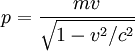andOne can verify that, in the non-relativistic limit, the relativistic values converge to the classical ones.

It is this requirement, and some "gedanken experiments" involving conversion between potential and kinetic energy, that lead to E=mc². These experiments involve some kind of object that isn't moving (though there might be internal motion that doesn't figure in the experiment) and therefore has no kinetic energy and only potential energy, turning into some things that have kinetic energy. The requirements of strict conservation of total momentum and total energy prove the equation.

Einstein's famous derivation involved light instead of tangible objects, but the result is the same.

## History of Experimental Verification

Because the change in mass arising from a given release of energy is so small (, which 1.11 x 10−17 kilograms per joule), it is essentially impossible to check this equation for normal processes. For example, a flashlight battery loses about 1 picogram of mass when it discharges, and the resultant atoms from the detonation of 1 kilogram of TNT weigh 47 nanograms less than the TNT. Even if all the particles of smoke and gas could be collected reliably, the difference couldn't be detected.

Measuring the effect requires process that release vastly more energy than ordinary chemical processes. The discovery of Radium and Polonium around 1898 gave a tantalizing hint that there were processes that released far more energy than chemical processes could account for. These elements continuously released measurable heat, and also glowed in the dark.

Einstein touched on this possibility in his original 1905 paper.

 “ It is not impossible that with bodies whose energy content is variable to a high degree (e.g. with radium salts) the theory may be successfully put to the test. ”
It would take more than a decade to develop an understanding of the nuclear process involved. The first thing that was required was accurate knowledge of atomic weights.

Atomic weights of the various elements were first measured, with accuracy of a few decimal places, by J. J. Berzelius in the late 1820s. This required extremely painstaking (for the time) measurements. The figures were refined to even more accuracy by J. A. R. Newlands in the 1860s. The values were accurate enough to clearly show the rather interesting property that the atomic weights were nearly integers, but not exactly so. The reason for this would turn out to be partly because of different isotopes (discovered by Frederick Soddy in 1913) and partly because of E=mc2.

In 1907 Rutherford determined that the "alpha" radiation from Radium was Helium. In 1911 he formulated the theory of the nucleus. In 1919 he demonstrated that nuclear transmutations could take place, such as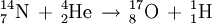The discovery of Radon, and much further investigation, revealed that the behavior of Radium was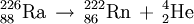andAccurate ways of measuring speed of a charged particle, by deflecting it in a magnetic field, had been developed by then, so that, by very painstaking observation and measurement, it was determined that the first alpha particle (Helium nucleus) had an energy of 4.78 MeV and the second an energy of 5.49 Mev. This confirmed E=mc2 up to the accuracy of the measurements. The equation, along with knowledge of isotope mixes, now explained why the atomic weights appearing in the periodic table were nearly integers, but not exactly so.

Around 1925, the development of the mass spectrograph, by Francis Aston, made it possible to measure atomic weights to extreme precision.

The 1932 Cockcroft-Walton experiment, described in more detail below, started to make the equation famous by confirming it, with reasonable accuracy, for an artificially induced nuclear reaction. (Confirming E=mc2 was not a goal of the experiment; it was an incidental consequence. The equation had already been known and understood for many years.)

In the decades since, nuclear transmutations have been performed, in particle accelerators, all over the periodic table, observing in detail the properties of various isotopes. These have confirmed E=mc2 with great precision. See Quantitative Analysis of Alpha Decay.

## The Rainville test

Perhaps the most precise direct empirical verification of E=mc2 was done in 2005 by Simon Rainville et. al. The article states that "Einstein's relationship is separately confirmed in two tests, which yield a combined result of 1−Δmc²/E=(−1.4±4.4)×10−7, indicating that it holds to a level of at least 0.00004%. To our knowledge, this is the most precise direct test of the famous equation yet described."

## The Cockcroft/Walton experiment

This experiment is not one of the ones usually cited as validating E=mc². That was not its goal. The generally accepted important tests of this equation are the measurements of alpha decay energies, described above.

In 1932 English physicist John Cockcroft and Irish physicist Ernest Walton performed the first artificial nuclear transmutation of nuclei, for which they were awarded the 1951 Nobel Prize in physics. The award was for "their pioneer work on the transmutation of atomic nuclei by artificially accelerated atomic particles."

Verifying E=mc² was not the goal of the experiment, and the Nobel prize was awarded for the transmutation itself, not any verification of the equation. This experiment could not have proved any general truth to the equation, since it was a test of just one specific reaction. But data from this experiment was consistent with the equation for the particular transmutation involved.

They bombarded Lithium atoms with protons having a kinetic energy less than 1 MeV. The result were two (slightly less heavy) alpha particles, for which the kinetic energy was measured as 17.3 MeV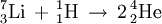The mass of the particles on the left hand side is 8.0263 amu, the mass on the right hand side only 8.0077 amu. The difference between this masses is .00186 amu, which results in the following back-of-an-envelope calculation: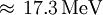Accurate measurements and detailed calculations allowed for verifying the theoretical values with an accuracy of ±0.5%. This was the first time a nucleus was artificially split, and thereby the first transmutation of elements using accelerated particles:

## A Famous Example -- Nuclear Fission of Uranium

For most types of physical interactions, the masses of the initial reactants and of the final products match so closely that it is essentially impossible to measure any difference. But for nuclear reactions, the difference is measurable. That difference is related to the energy absorbed or released, described by the equation E=mc². (The equation applies to all interactions; the fact that nuclear interactions are the only ones for which the mass difference is measurable has led people to believe, wrongly, that E=mc² applies only to nuclear interactions.)

The Theory of Relativity played no role in this work, but proponents later tried to retrofit the theory to the data in order to explain the explain the observed mass changes. Here is the most famous example of the mass change.

Nuclear fission, which is the basis for nuclear energy, was discovered in experiments by Otto Hahn and Fritz Strassman, and analyzed by Lise Meitner, in 1938.

There are a great number of decay paths of Uranium fission that figured in this experiment. The result element that most caught their attention was Barium, because it was chemically related to the Radium that they were expecting. One of the fission paths may have been this:

235U → 140Xe + 91Sr + 4n

(The Xenon decayed within about a minute to 140Ba. They were searching for the chemical signature of Barium.)

The masses of the particles are:

Substance 235U 140Xe 91Sr 4 neutrons
Number of protons 92 54 38 0
Number of neutrons 235 140 91 4
Number of electrons 92 54 38 0
Mass 235.04393 139.92164 90.910203 4.03466

The mass of the Uranium atom is 235.04393, and the sum of the masses of the products is 234.866503. The difference is .177427 amu, or, using the E=mc² equation, 165 million electron volts. (The generally accepted value for the total energy released by Uranium fission, including secondary decays, is about 200 million electron volts.)

The insight that the conversion from Uranium to Barium was caused by complete fission of the atom was made by Lise Meitner in December, 1938. She had the approximate "mass defect" quantities memorized, and so she worked out in her head, using the E=mc² equation, that there would be this enormous release of energy. This release was observed shortly thereafter, and the result is nuclear power and nuclear weapons.

## A Topical Example: Speed of Extremely Energetic Neutrinos

Here is another example of the use of this formula in physics calculations. In 2011 there were reports that high-energy neutrinos had been observed traveling at a speed faster than the speed of light in an experiment at the Gran Sasso laboratory in Italy. Specifically, they seemed to have arrived at the detector 60 nanoseconds faster than light would have. Relativity doesn't allow that, and, since neutrinos have nonzero (but incredibly tiny) mass, they aren't even supposed to travel at the speed of light.

The mass of a neutrino is about 0.44x10−36kilograms. (Normally all of these things are measured in more convenient units such as Giga-electron-Volts, but that makes implicit use of E=mc2. If we don't accept that, we have to do the calculations under classical physics, using SI (meter/kilogram/second) units.) The neutrinos were accelerated to an energy of about 17GeV, or .27x10−8Joules. If one did not accept relativity and had to use classical physics and the classical formula, one would get v=110x1012 meters per second. This is about 370,000 times the speed of light, something that scientists would certainly have noticed. In fact, with special relativity, the speed is just under the speed of light, such that the neutrinos should be received at the detector about .26x10−24 seconds (.26 yoctoseconds) later than the speed of light itself. This is far too small to measure—15 orders of magnitude smaller than the resolution of the GPS signals in the experiment.

Later reports started to resolve the mystery, and it is now accepted that the neutrinos behaved properly. But a BBC reporter made the incorrect statement that the neutrinos travelled at precisely light speed. This was a simple misstatement, by .26 yoctoseconds.

The issue was discussed at length at Conservapedia.

## Deducing the Equation From Empirical Observation

While the equation was historically developed on theoretical grounds as an inevitable consequence of special relativity, it is possible to deduce it purely from empirical observation.

So, for the purposes of this section, imagine that one is in the era of "classical physics"; prior to 1900 or so. Relativity has not been invented, but, inexplicably, nuclear physics has. Imagine that the phenomena of radioactivity and nuclear fission have been observed, without any knowledge of relativity.

A well-accepted physical law of classical physics was the law of conservation of mass. This was not easy to deduce. It required careful analysis of such phenomena as combustion, in the 1700s, to eliminate the various confounding sub-phenomena that made the law difficult to see. But, by 1900, the law was well established:

• In all interactions, mass is precisely conserved.

For example, the mass of a TNT molecule is 227.1311 Daltons, or 227.1311 g/mol, which is, for all practical purposes, the same as the mass of its constituent Carbon, Hydrogen, Nitrogen, and Oxygen atoms. It is essentially impossible to measure the difference. The principle of conservation of mass is upheld.

But when nuclear phenomena are discovered, we notice something different. The masses of the result particles after an event (e.g. alpha decay, nuclear fission, or artificial transmutation) is measurably less than the masses of the original particle(s). With the invention of the mass spectrometer around 1920, it became possible to measure atomic weights of various isotopes with great precision.

Radium-226 decays into Radon-222 by emission of an alpha particle with an energy of 4.78 MeV.

1 kg of Radium-226 =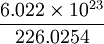atoms. (The numerator is Avogadro's number, and the denominator is the atomic weight of Radium-226.) This is 2.6643647 * 1024 atoms.

That number of Radon-222 atoms has mass .98226836 kg. That number of alpha particles has mass .01770863 kg. The mass lost is .00002301 kg.

Each emitted alpha particle has energy of 4.78 MeV, or 4.78 * .1602 * 10−18 Joules. The total alpha energy from the decay of 1 kg of radium is 2.04 * 1012 Joules.

Also, Radon-222 decays into Polonium-218 by emission of an alpha particle with an energy of 5.49 MeV.

1 kg of Radon-222 =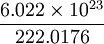atoms. This is 2.7124611 * 1024 atoms.

That number of Polonium-218 atoms has mass .98194467 kg. That number of alpha particles has mass .01802830 kg.

The mass lost is .00002703 kg.

Each emitted alpha particle has energy of 5.49 MeV. The total alpha energy from the decay of 1 kg of polonium is 2.39 * 1012 Joules.

It looks as thought we have to rewrite the law of conservation of mass:

• In all "ordinary" interactions, mass is precisely conserved.
• In nuclear interactions, there is a small but measurable loss of mass.
By the way, we can clearly see that atomic weights of pure isotopes are not integers, and that it has something to do with the energy released by nuclear disintegration. In retrospect, the formula E=mc² explains the non-integer character of atomic weights.

Making special cases like this is unsatisfactory, of course.

We do this for a few other interactions, including the explosion of TNT. This would include the Lithium-plus-Hydrogen and Uranium fission phenomena described above. We won't bother with the details. As observational scientists, we look for patterns in the behavior of nature. We make a table:

interaction energy released per kg, Joules mass lost per kg of original substance, kg
explosion of TNT 4.184 * 106 seems to be zero
alpha decay of Ra-226 2.04 * 1012 .00002301 kg
alpha decay of Rn-222 2.39 * 1012 .00002703 kg

We plot these, and a few others, not shown, on graph paper, and find to our amazement that the relationship is linear.

For Ra, m/E = .112794118 E-16 For Po, m/E = .113096234 E-16

If this is linear, the mass defect for TNT would have been .47 * 10−10. We couldn't possibly have measured this.

So we can rewrite the rule for conservation of mass in a more satisfactory way:

• In all interactions, there is a loss of mass, equal to about .113 * 10-16 kg per Joule of energy released.

What we thought was exact conservation is just very nearly exact, and we hadn't been able to measure it before.

But maybe there's more. This constant has dimensions of kilograms per Joule. From high-school physics, we know that that is seconds squared divided by meters squared. That is, it is the reciprocal of the square of a velocity. We calculate that velocity. It is about 2.97 * 108 meters per second. Very close to the speed of light! Very interesting! (The calculations above were not extremely precise. The formula has been verified with great precision, but not here.)

We don't understand why (that will have to wait for the invention of relativity), but we can formulate a hypothesis:

• In all interactions, there is a loss of mass, equal totimes the amount of energy released.

We don't have to give the units any more, since everything is now dimensionally correct.

There is a very interesting analogy with the discovery of Maxwell's Equations. Maxwell found an interesting relationship involving the fundamental constantsand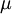appearing in his equations. Specifically,has the dimensions of seconds squared divided by meters squared, and that: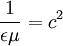where "c" was the known velocity of light. He also showed that his equations predict electromagnetic waves, propagating at that speed.